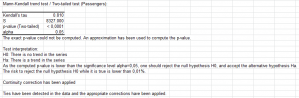# Mann-Kendall Trend Tests

The Mann-Kendall trend test is a non parametric way to detect a trend in a series of values. Available in Excel using the XLSTAT statistical software.## What is the Mann-Kendall test

Mann-Kendall trend test is a nonparametric test used to identify a trend in a series, even if there is a seasonal component in the series.

## Mann-Kendall test history

This test is the result of the development of the nonparametric trend test first proposed by Mann (1945). This test was further studied by Kendall (1975) and improved by Hirsch et al (1982, 1984) who allowed to take into account a seasonality.

## Mann-Kendall trend test hypotheses

The null hypothesis H0 for these tests is that there is no trend in the series.

The three alternative hypotheses are that there is a negative, non-null, or positive trend.

The Mann-Kendall tests are based on the calculation of Kendall's tau measure of association between two samples, which is itself based on the ranks with the samples. The computations assume that the observations are independent.

XLSTAT allows taking into account and removing the effect of autocorrelations. Sen's slope is computed if you request to take into account the autocorrelation(s)

## Mann-Kendall trend test

In the particular case of the trend test, the first series is an increasing time indicator generated automatically for which ranks are obvious, which simplifies the calculations.

To calculate the p-value of this test, XLSTAT can calculate, as in the case of the Kendall tau test, an exact p-value if there are no ties in the series and if the sample size is less than 50. If an exact calculation is not possible, a normal approximation is used, for which a correction for continuity is optional but recommended.

## Seasonal Mann-Kendall test

In the case of seasonal Mann-Kendall test, we take into account the seasonality of the series. This means that for monthly data with seasonality of 12 months, one will not try to find out if there is a trend in the overall series, but if from one month of January to another, and from one month February and another, and so on, there is a trend.

For this test, we first calculate all Kendall's tau for each season, then calculate an average Kendall’s tau. The variance of the statistic can be calculated assuming that the series are independent (eg values of January and February are independent) or dependent, which requires the calculation of a covariance. XLSTAT allows both (serial dependence or not).

To calculate the p-value of this test, XLSTAT uses a normal approximation to the distribution of the average Kendall tau. A continuity correction can be used.### analyze your data with xlstat

14-day free trial

Included in

Related features